# 1. Find the volume of the solid described by the inequality Vz? + y2 <2< 2.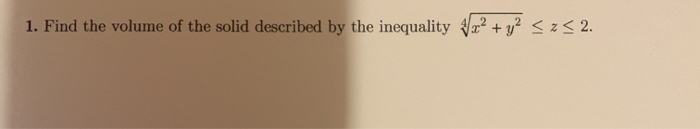1. Find the volume of the solid described by the inequality Vz? + y2 <2< 2.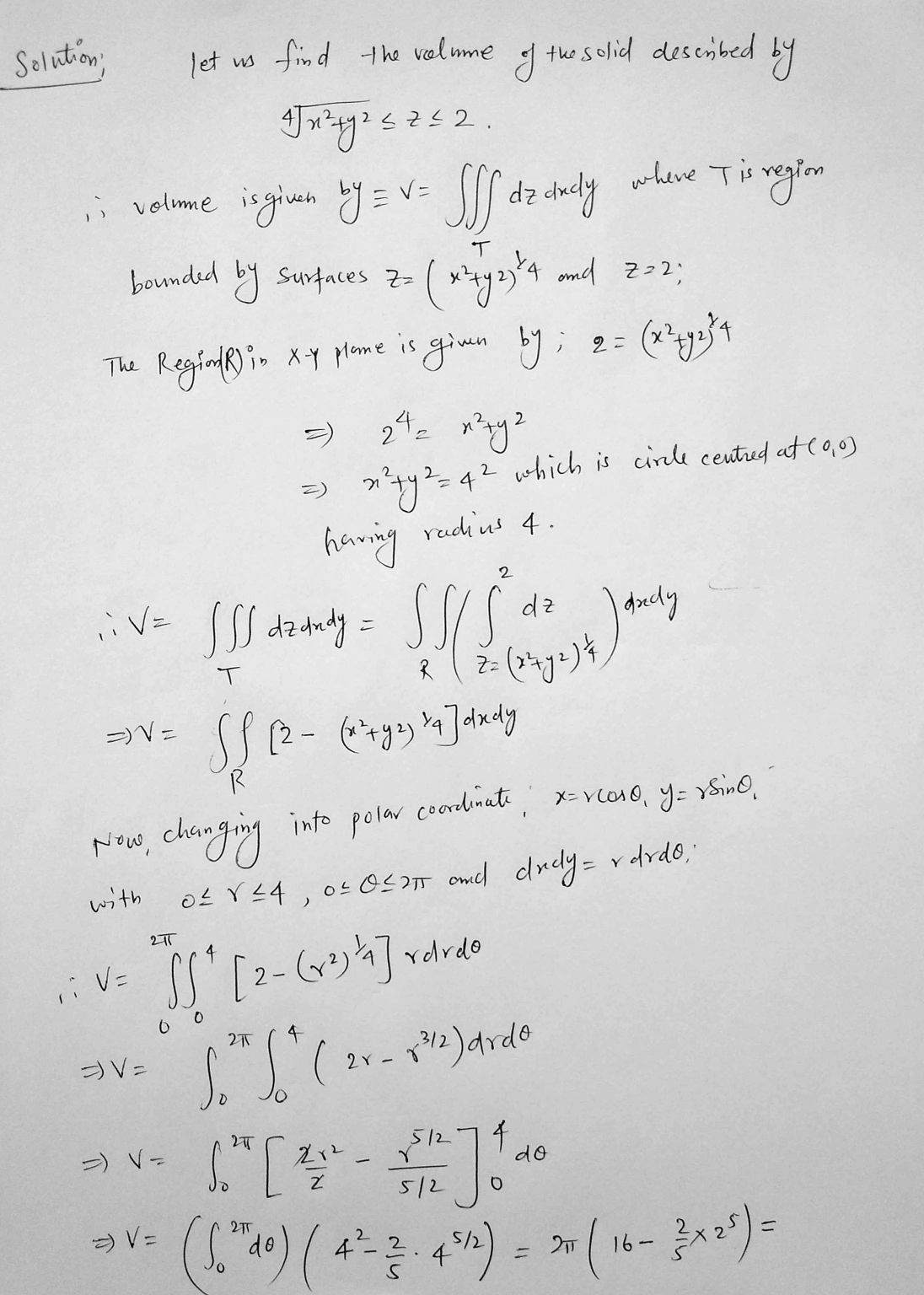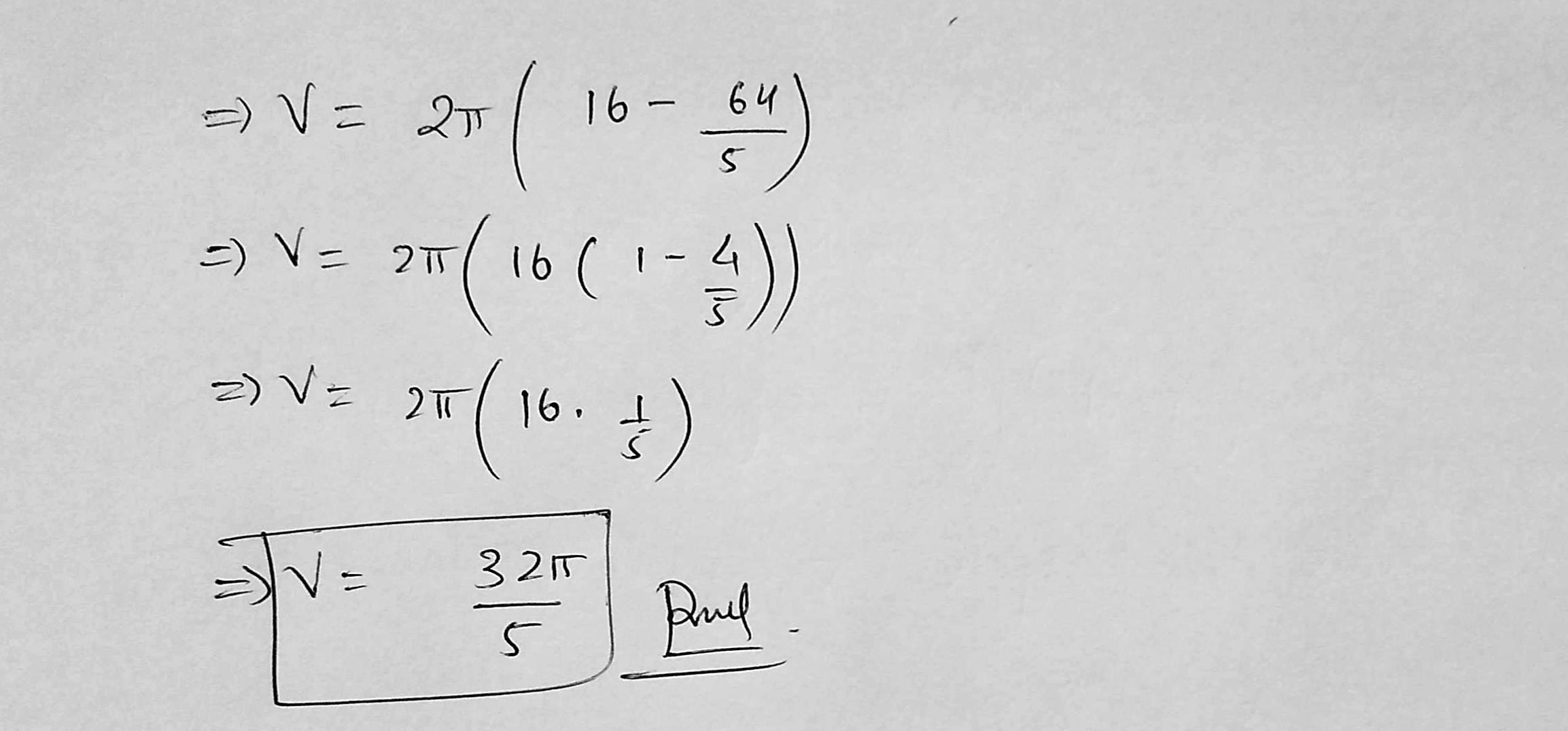##### Add Answer of: 1. Find the volume of the solid described by the inequality Vz? + y2 <2< 2.
Similar Homework Help Questions
• ### Solve the inequality 22 +2 - 2 22 - 5.0 + 6 <0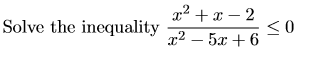Solve the inequality 22 +2 - 2 22 - 5.0 + 6 <0

• ### Let Yı, Y2, ...,Yn be an iid sample from a population distribution described by the pdf...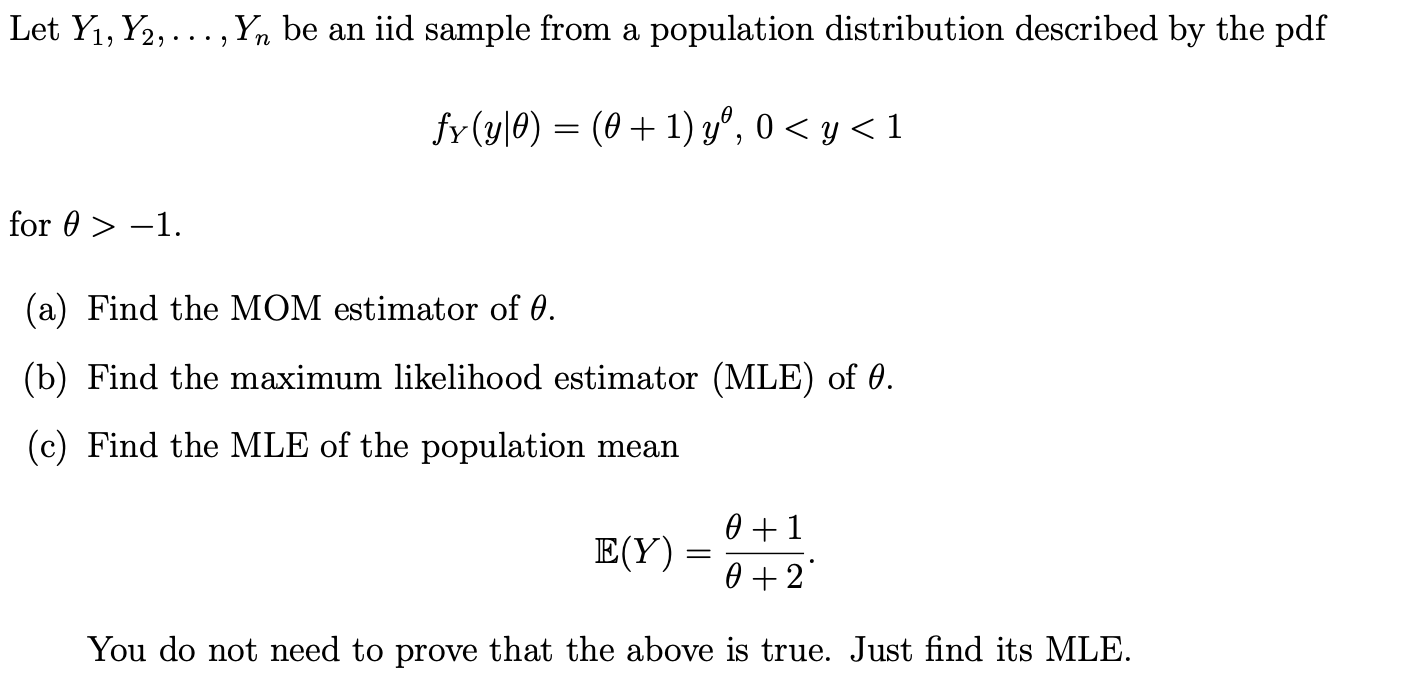Let Yı, Y2, ...,Yn be an iid sample from a population distribution described by the pdf fy(y|0) = (@+ 1) yº, o<y<1 for 0> - -1. (a) Find the MOM estimator of 0. (b) Find the maximum likelihood estimator (MLE) of 0. (c) Find the MLE of the population mean E(Y) = 0 +1 0 + 2 You do not need to prove that the above is true. Just find its MLE.

• ### Compute the volume SSSx 1 dV where X is the solid defined by x2 + y2...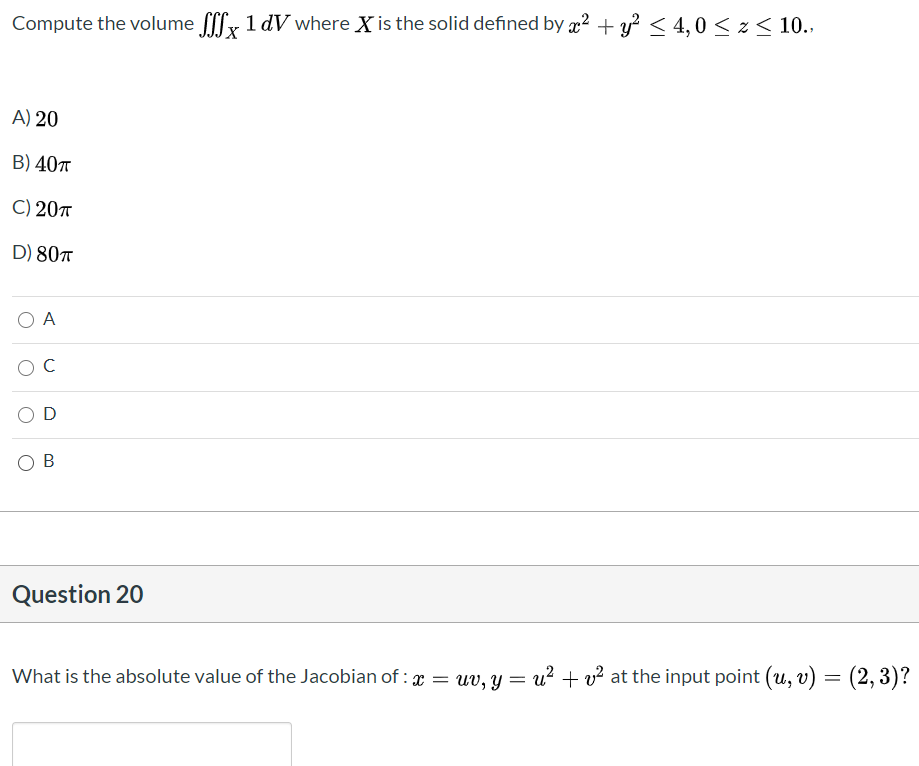Compute the volume SSSx 1 dV where X is the solid defined by x2 + y2 < 4,0 Sz<10., A) 20 B) 407 C) 201 D) 801 ОА ОС OD OB Question 20 What is the absolute value of the Jacobian of : x = uv, y = u2 + v2 at the input point (u, v) = (2, 3)?

• ### 5. (15 points) Write the integral representing the area of the region a< x2 + y2...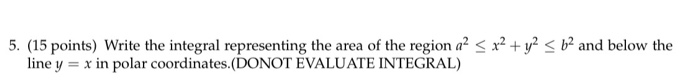5. (15 points) Write the integral representing the area of the region a< x2 + y2 < b> and below the line y = x in polar coordinates.(DONOT EVALUATE INTEGRAL)

• ### 4. Show that the sequence defined by a=2 An+1- 3-an satisfies () < an < 2...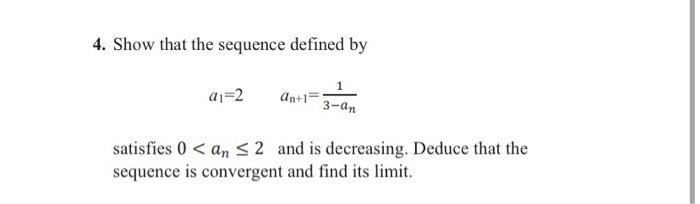4. Show that the sequence defined by a=2 An+1- 3-an satisfies () < an < 2 and is decreasing. Deduce that the sequence is convergent and find its limit.

• ### Provided N(0, 1) and without using the LSND program, find P( - 2 <3 <0) Provided...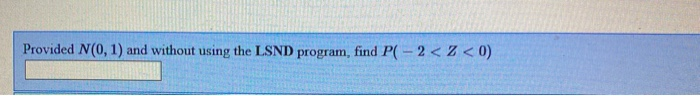Provided N(0, 1) and without using the LSND program, find P( - 2 <3 <0) Provided N(0, 1) and without using the LSND program, find P(Z < 2). Provided N(0, 1) and without using the LSND program, find P(Z <OOR Z > 2). Message instructor about this question Provided N(0, 1) and without using the LSND program, find P(-1<2<3). 0.84 Message instructor about this question

• ### 1. Let x, a € R. Prove that if a <a, then -a < x <a.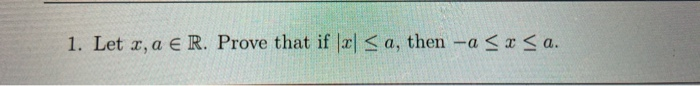1. Let x, a € R. Prove that if a <a, then -a < x <a.

• ### Solve the follwing rational inequality: 2 – 3 < 0 x + 1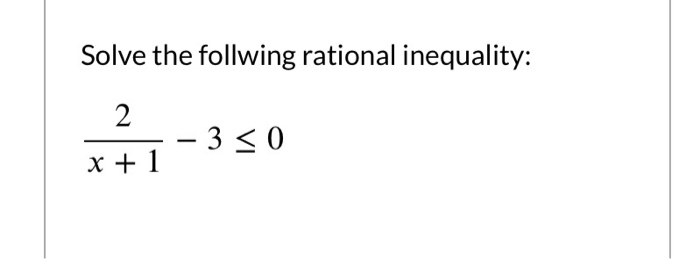Solve the follwing rational inequality: 2 – 3 < 0 x + 1

• ### 9) Find the absolute maxima and minima of the function f(x,y) = x2 + xy +...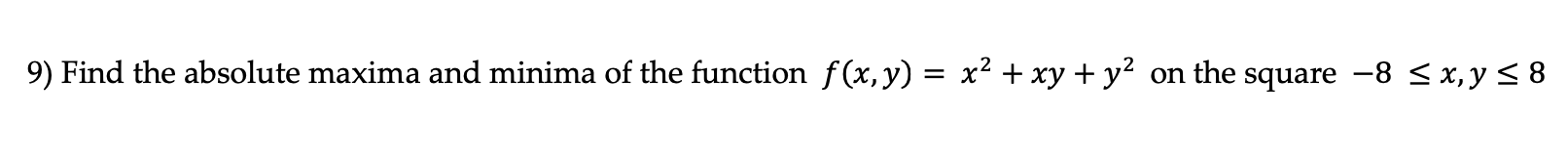9) Find the absolute maxima and minima of the function f(x,y) = x2 + xy + y2 on the square -8 < x,y 5 8

• ### Question 19 1 pts Compute the volume SSSx 1dV where X is the solid defined by...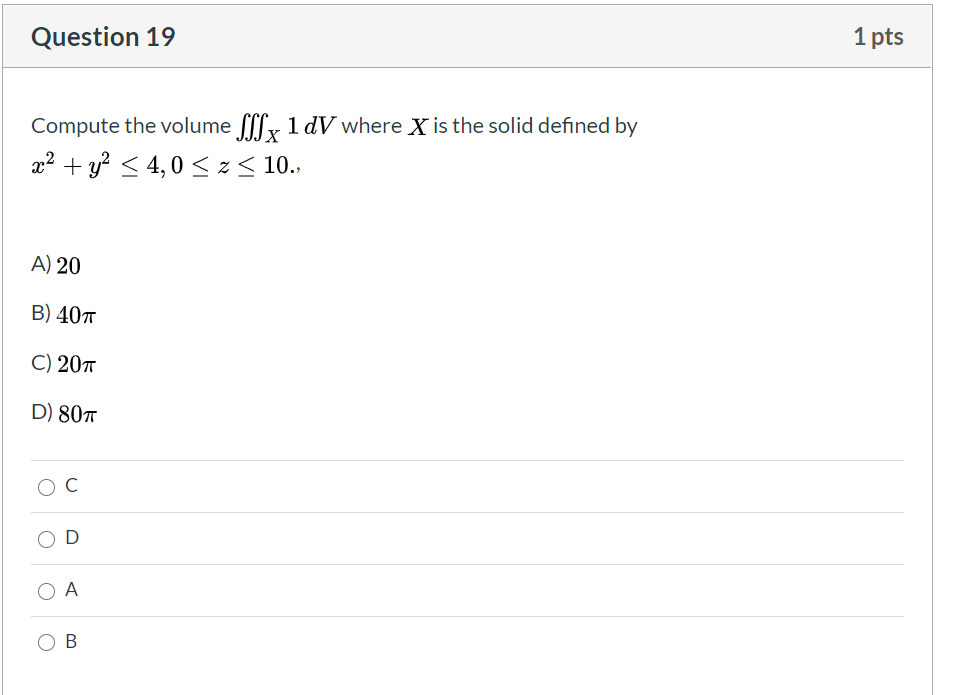Question 19 1 pts Compute the volume SSSx 1dV where X is the solid defined by x2 + y2 < 4,0 <z10., A) 20 B) 407 C) 207 D) 807 C o ОА OB

Free Homework App